• +91 9971497814
• info@interviewmaterial.com

# Chapter 2- Inverse Trigonometric Functions Ex-2.2 Interview Questions Answers

### Related Subjects

Question 1 : Prove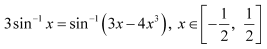Answer 1 : To prove:Let x = sinθ.Then,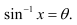We have,

R.H.S.=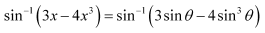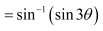= 3θ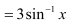=L.H.S.

Question 2 : Prove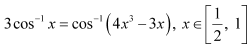Answer 2 : To prove:Let x = cosθ.Then, cos−1 x =θ.

We have,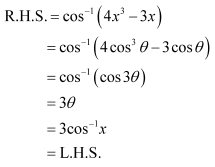Question 3 : Prove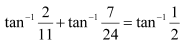Answer 3 : To prove: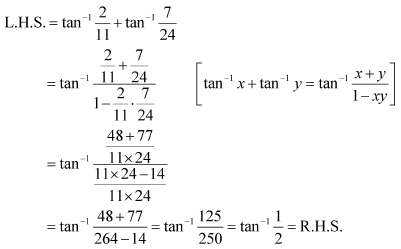Question 4 : Prove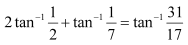Answer 4 : To prove: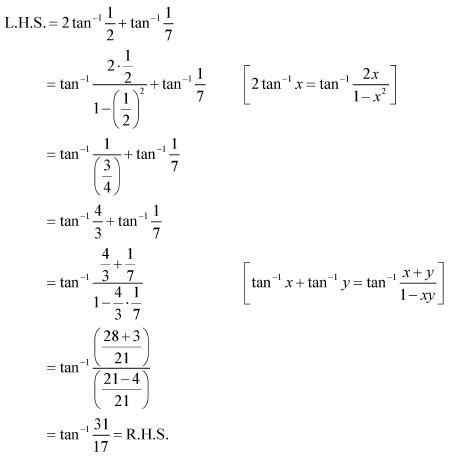Question 5 :

Write the function in thesimplest form: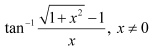Answer 5 :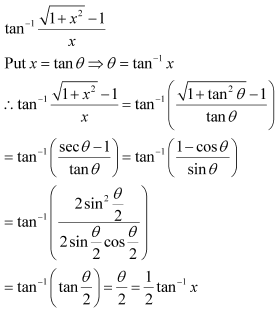Question 6 :

Write the function in thesimplest form: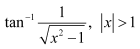Answer 6 :Put x =cosec θ  θ =cosec−1 x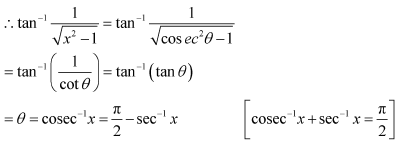Question 7 :

Write the function in thesimplest form: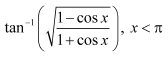Answer 7 :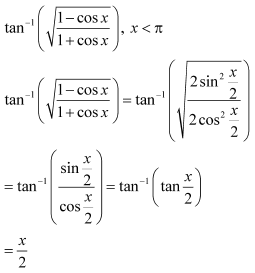Question 8 :

Write the function in thesimplest form: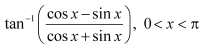Answer 8 :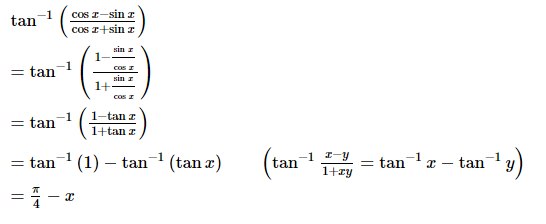Question 9 :

Write the function in thesimplest form: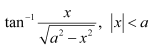Answer 9 :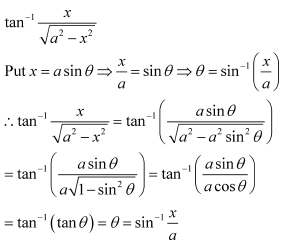Question 10 :

Write the function in thesimplest form: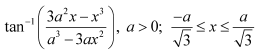Answer 10 :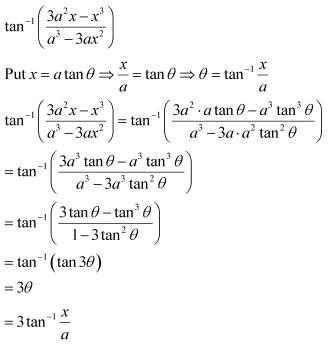Todays Deals### Chapter 2- Inverse Trigonometric Functions Ex-2.2 Contributorskrishan

Name:
Email:

# Latest News# 9000 interview questions in different categories